# Key Words for Inequalities Less Than Greater Than

• Slides: 13Key Words for Inequalities Less Than < Greater Than > Is Less Than Is Fewer Than Greater Than More Than Exceeds Less Than or Equal To ≤ Greater Than or Equal To ≥ Is Less Than or Equal To Is No More Than Is At Most Is Greater Than or Equal To Is No Less Than Is At Least≥ ≤ < > Children 10 and under eat free! X = age of child X ? 10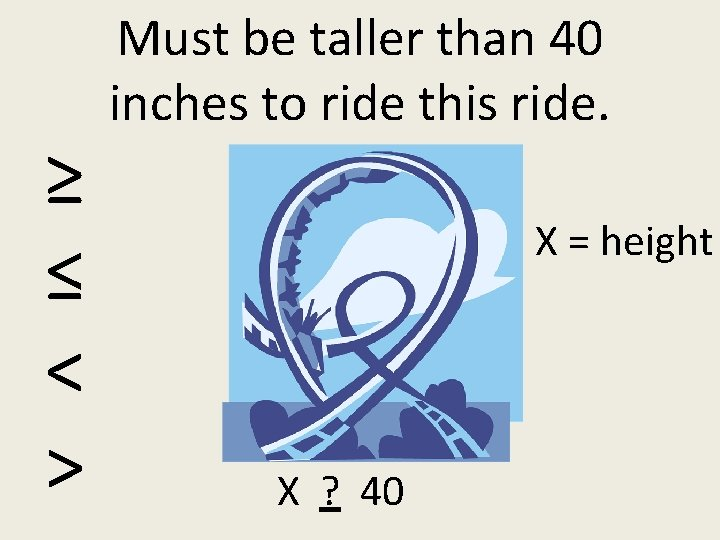≥ ≤ < > Must be taller than 40 inches to ride this ride. X = height X ? 40X > 40 X ≤ 65 X ≤ 10 These are called inequalities!Write an inequality for this information. Your age is less than 20 years. (Let x represent your age).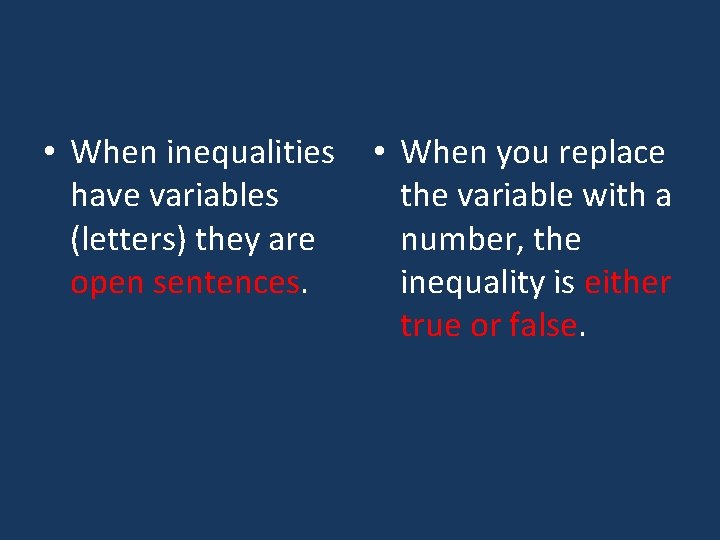• When inequalities have variables (letters) they are open sentences. • When you replace the variable with a number, the inequality is either true or false.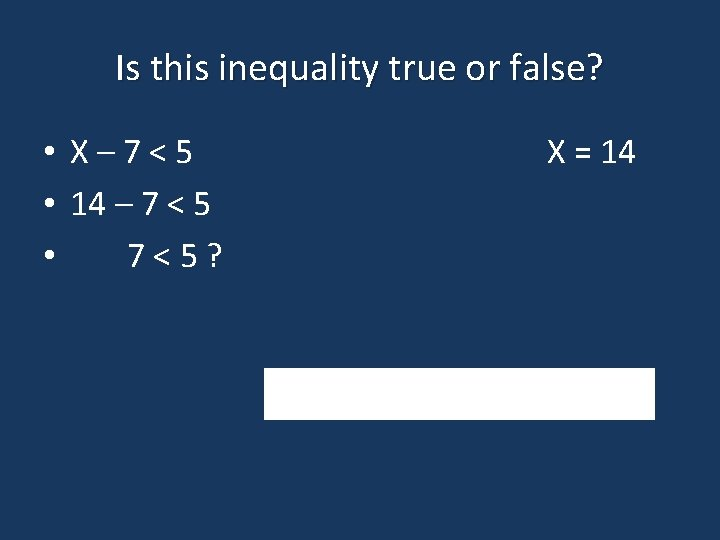Is this inequality true or false? • X– 7<5 • 14 – 7 < 5 • 7<5? X = 14YOU TRY! Is this inequality true or false? • 25 + X > 15 X = 10YOU TRY! Is this inequality true or false? • 25 - X ≥ 15 X = 10Inequalities can be graphed on a number line. “X” represents a number.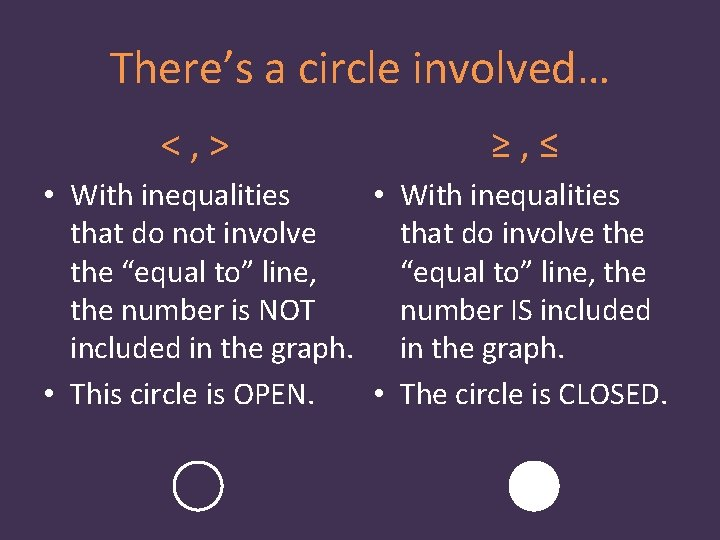There’s a circle involved… <, > ≥, ≤ • With inequalities that do not involve that do involve the “equal to” line, the number is NOT number IS included in the graph. • This circle is OPEN. • The circle is CLOSED.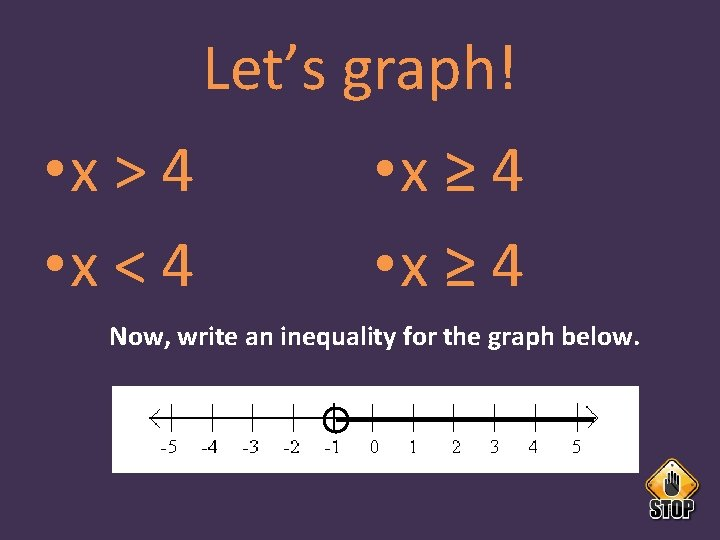Let’s graph! • x > 4 • x < 4 • x ≥ 4 Now, write an inequality for the graph below.